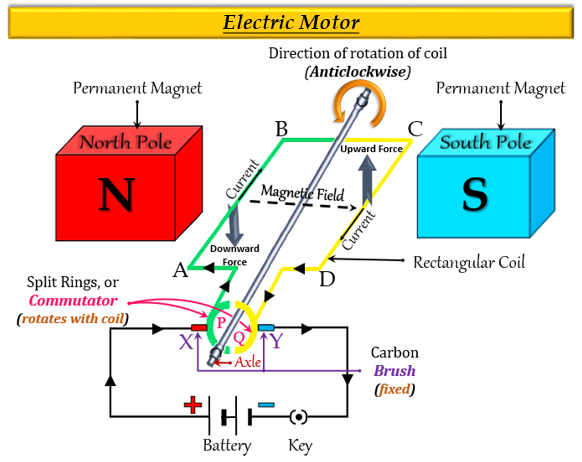# (a) State what would happen to the direction of rotation of a motor if: (i) the current were reversed (ii) the magnetic field were reversed (iii) both current and magnetic field were reversed simultaneously. (b) In what ways can a motor be made more powerful?

(a) (i) If the current were reversed, then the direction of rotation of a motor would be reversed.
(ii) If the magnetic field were reversed, then the direction of rotation of a motor would be reversed.
(iii) If both current and the magnetic field were reversed simultaneously then the direction of rotation of a motor would remain unchanged.

Explanation
Upon reversing either the current or the magnetic field, the direction of the forces acting on the sides of the coil is reversed, and the rotation of the motor gets reversed.
If both these factors are reversed simultaneously, the motor will continue to rotate in the same direction.(b) A motor can be made more powerful in the following ways:
1. Winding the coil on to a soft iron core.
2. Increasing the number of turns of insulated copper wire of the coil.
3. Using a powerful electromagnet in place of a permanent magnet.

Updated on: 10-Oct-2022

174 Views